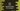# Dart program to find the absolute value of a number## How to find the absolute value of a number in Dart:

Dart Number class provides a method called abs that can be used to find the absolute value of a number. We can use this method to find the absolute value of a number. In this post, we will learn how to use Number.abs with an example.

### Number.abs in Dart:

Number.abs method is defined in Number class in Dart. It is defined as below:

``abs() -> num``

It returns the absolute value of the number.

### Example of abs():

Let’s take a look at the below dart program:

``````void main() {
var number_one = 10.6;
var number_two = -10.6;
var number_three = 11;
var number_four = -11;

print(number_one.abs());
print(number_two.abs());
print(number_three.abs());
print(number_four.abs());
}``````

If you run it, it will give the below output:

``````10.6
10.6
11
11``````